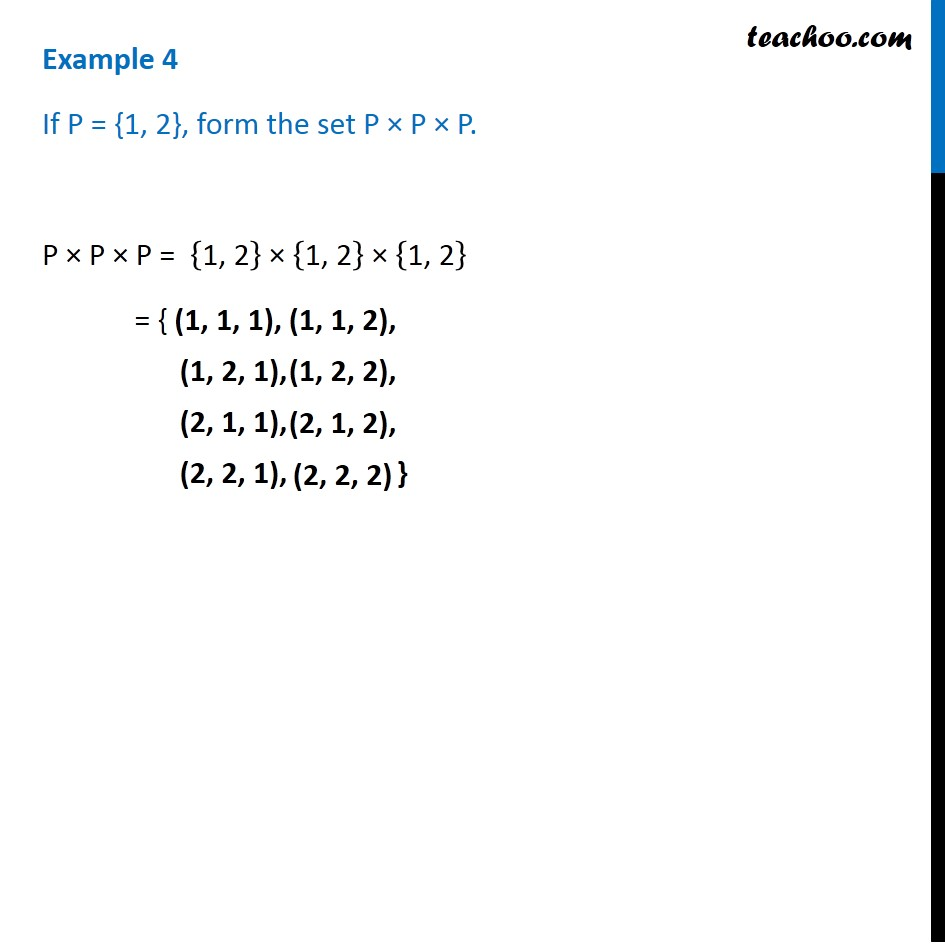Learn All Concepts of Chapter 2 Class 11 Relations and Function - FREE. Check - Relation and Function Class 11 - All Concepts1. Chapter 2 Class 11 Relations and Functions
2. Serial order wise
3. Examples

Transcript

Example 4 If P = {1, 2}, form the set P × P × P. P × P × P = {"1, 2" } × {"1, 2" } × {"1, 2" } = { (1, 1, 1), (1, 1, 2), (1, 2, 1), (1, 2, 2), (2, 2, 1), (2, 2, 2)

Examples